# MCAT Physical : Partial Pressure

## Example Questions

### Example Question #1 : Partial Pressure

Which of the following is a direct application of Henry's law?

Air pressure in the lungs

Volume in lung capacity

Tract system from nostrils to alveoli

Viscosity of the fluid in the lungs

Gas exchange in the lungs

Gas exchange in the lungs

Explanation:

Henry's Law directly applies to gas exchange in the lungs, as it states that the amount of dissolved gas in a liquid are proportional to the solubility of the gas in the liquid. As oxygen is dissolved in the bloodstream, it is able to diffuse into the air of the lungs depending upon intrapleural pressure of that particular gas. Essentially, Henry's law dictates how readily oxygen will cross the alveolar epithelium.

### Example Question #2 : Partial Pressure

A container has three moles of gas A, three moles of gas B, and six moles of gas C. The total pressure of the system is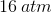. The partial pressure of gas B is __________ less than the partial pressure of gas C.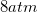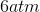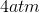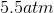Explanation:

To solve this question you need to know the Dalton’s Law of partial pressure:In this equation,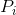is the partial pressure of the gas,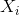is the mole fraction of the gas, and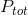is the total pressure of the system. You also need to know the definition of mole fraction: moles of a compound divided by total moles in the system.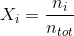This question requires us to calculate the partial pressure of both gas B and gas C. First, let’s find the partial pressure of gas B. The mole fraction of gas B is: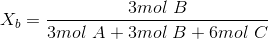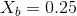We are given the total pressure of the system. Using the calculated mole fraction, we can solve for the partial pressure of gas B: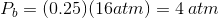Similarly, we can find the partial pressure of gas C. The mole fraction of gas C is: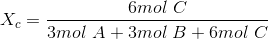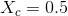The mole fraction times the total pressure will give the partial pressure of gas C: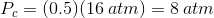Finally, find the difference between the partial pressures of the two gases.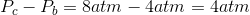The partial pressure of gas B isless than the partial pressure of gas C.

### Example Question #3 : Partial Pressure

The molecular weight of gas A is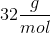.

Gas A is in a mixture with four other gases and has a partial pressure of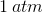. The other four gases have partial pressures of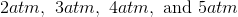respectively.

What is the mass of gas A in this mixture?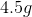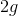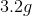Cannot be determined from the given information

Cannot be determined from the given information

Explanation:

Recall Dalton’s Law of partial pressure: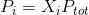In this equation,is the partial pressure of the gas,is the mole fraction of the gas, andis the total pressure of the system. The mole fraction,, is defined as the moles of a compound divided by the total moles in the system:From the given information, we can solve for mole fraction of gas A using the partial pressure of gas A and the total pressure of the system.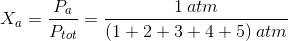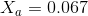To find the mass of gas A we need to find the moles of gas A in the mixture and multiply it by the molecular weight of gas A; however, the information given in this question only allows us to solve for the mole fraction of gas A, or percentage of gas A in the system. To convert mole fraction to moles we would need to know the total number of moles in the mixture; therefore, the mass of gas A cannot be determined.

### Example Question #1 : Partial Pressure

A container with a total pressure (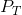) of 3atm holds 0.80mol of oxygen and 0.20mol of nitrogen. What is the partial pressure of oxygen in this container?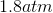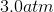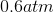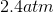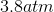Explanation:

Partial pressure is given by the mole fraction of a gas multiplied by the total pressure in the container. Mole fraction is equal to the moles of a given compound divided by the total moles.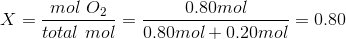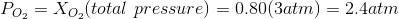This is an application of Dalton's Law.

### Example Question #1 : Partial Pressure

Which of the following is false regarding partial pressure?

Partial pressure signifies the pressure of a gas in a system at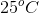Partial pressure can be calculated if you know the mole fraction of the gas and the total pressure of the system

Dalton’s Law of partial pressure is only valid for a mixture of inert gases

In Earth’s atmosphere, nitrogen has the largest partial pressure

Partial pressure signifies the pressure of a gas in a system atExplanation:

Partial pressure of a gas is defined as the pressure of the gas in a mixture of gases. It is calculated by using Dalton’s Law of partial pressure, which states that the partial pressure is equal to the product of the mole fraction of the gas and the total pressure of the system.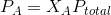One of the assumptions of this law is that the gases are independent and do not chemically react with each other. This means that the gases in the system have to be inert. If the gases were reactive with each other, then Dalton’s Law would become invalid. Recall that Earth’s atmosphere contains about 78% nitrogen, 21% oxygen, and 1% all other gases; therefore, nitrogen has the largest partial pressure in the atmosphere because it has the greatest mole fraction.

Partial pressure does signify the pressure of a gas in a mixture of gases, but it is only valid for the temperature of the system. The partial pressure of the gas will be different for different temperatures. This occurs because pressure is dependent on temperature. Changing the temperature will not alter the mole fraction (amount of gas); however, it will alter the total pressure which will subsequently alter the partial pressure of the gas according to Dalton's Law.